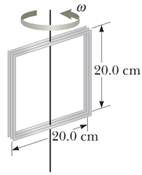CATEGORIES:

1.A 100 turn wire coil of 0.040 m2 rotates about axis at 1500 rev/min, as indicated in the figure. The horizontal component of the Earth’s magnetic field at the location of the loop is 2.00 × 10-15 T. Calculate the maximum emf induced in the coil by the Earth’s field.

2.Considerable scientific work is currently underway to determine whether weak oscillating magnetic fields such as those found near outdoor electric power lines can affect human health. One study indicated that a magnetic field of magnitude 1.00 mT, oscillating at 60.0 Hz, might stimulate red blood cells to become cancerous. If the diameter of a red blood cell is 8.00 mT, determine the maximum emf that can be generated around the perimeter of the cell.

3. An AC generator consists of 8 turns of wire, each having area A = 0.0900 m2, with a total resistance of 12.0 W. The loop rotates in a magnetic field of 0.500 T at a constant frequency of 60.0 Hz. (a) Find the maximum induced emf and rms-emf. (b) What is the maximum induced current and rms-current? (c) Determine the induced emf and current as a function of time. (d) What maximum torque must be applied to keep the coil turning?

4. An AC generator is to have a maximum output of 301 V. Each coil has an area of 0.100 m2 and a resistance of 16.0 W and rotates in a magnetic field of 0.600 T with a frequency of 40.0 Hz. (a) How many turns of wire should the coil have to produce the desired emf? (b) Find the maximum current induced in the coil. (c) Determine the induced emf as a function of time.

5. A coil of 10.0 turns is in the shape of an ellipse having a major axis of 10.0 cm and a minor axis of 4.00 cm. The coil rotates at 100 rpm in a region in which the magnitude of the Earth’s magnetic field is 55.0 mT. What is the maximum voltage induced in the coil if the axis of rotation of the coil is along its major axis and is aligned (a) perpendicular to the Earth’s magnetic field and (b) parallel to the Earth’s magnetic field? (Note that the area of an ellipse is given by A = pab, where a is the length of the semimajor axis and b is the length of the semiminor axis.)

AC-Circuit

1. 1.26 x 10-12 V;

2. 1.90 x 10-11 V;

3. (a) 136 V, 96.2 V, (b) 11.3 A, 7.99 A, (c) Ɛ(t) =136[sin(377t)] V, I(t) = 11.3[sin(377t)] A, (d) 4.06 Nm;

4. (a) n = 20 (b) 18.8 A, (c) Ɛ(t) =301[sin(251t)] V;

5. (a) 18.1 mV, (b) 0 V.

Date: 2015-01-29; view: 2453

 <== previous page | next page ==> Introduction to Kinematics and Calculus in Physics | UNITS and DIMENSIONS NU-CPS Physics 2014-15
doclecture.net - lectures - 2014-2023 year. Copyright infringement or personal data (0.012 sec.)